Java中lambda表达式详解

为什么使用lambda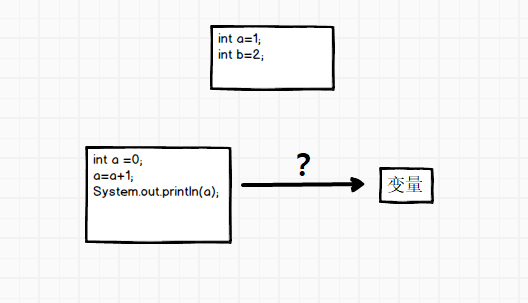var t=function()
{
int a=1;
a=a+1;
}



什么是lambda


interface eat
{
void eatFood();
}

public static void main(String[] args)
{

eat e = () -> System.out.printf("hello\n");
e.eatFood();

eat e1 = new eat()
{
@Override
public void eatFood()
{
System.out.printf("anoymous class\n");
}
};
e1.eatFood();
}



lamda的优点

• 代码缩减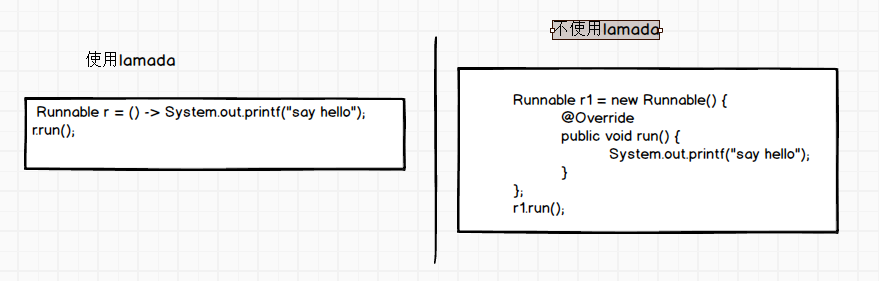• Option的使用简化代码

假如我们有个方法，能够产生一个Option对象std


Option<Person> std=getStudent();


1. 是否为空的判断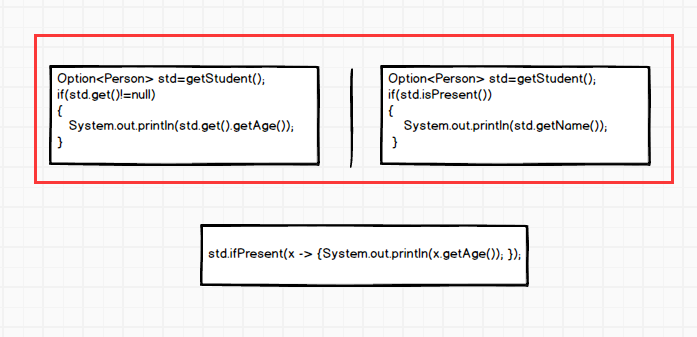2. 返回不为空的对象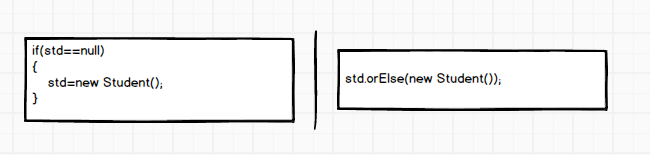3. 多重if else的简化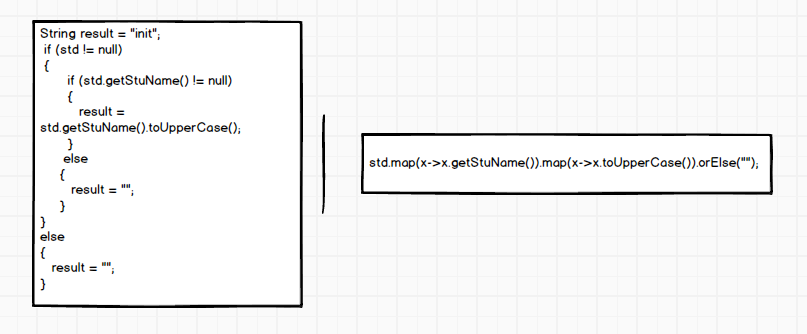函数式接口

//这个是函数式接口
interface eat
{
void eatFood();
}

//这个不是是函数式接口
interface eat
{
default void eatFood()
{
System.out.println("hello");
};
}

//这个是一个函数式接口
interface eat
{
void eatFood();
String toString();
}




//此处会报编译错误
@FunctionalInterface
interface eat
{
default void eatFood()
{
System.out.println("hello");
};
}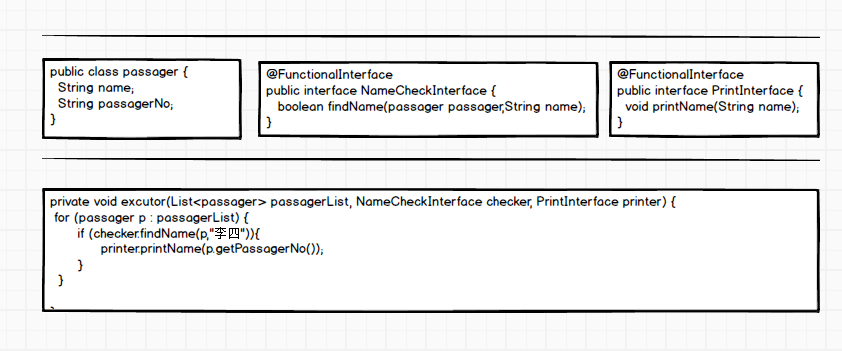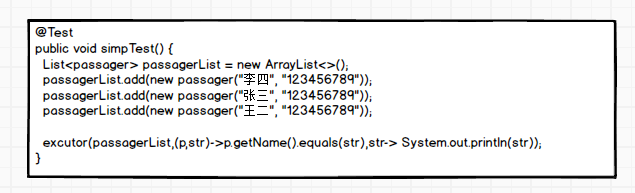@FunctionalInterface
public interface NameCheckInterface<T,T1,T2>
{
T2 findName(T passager,T1 name);
}

@FunctionalInterface
public interface PrintInterface<T>
{
void printName(T name);
}

private void excutor(List<passager> passagerList, NameCheckInterface<Boolean,passager,String> checker, PrintInterface<String> printer)
{
for (passager p : passagerList) {
if (checker.findName(p,"李四")){
printer.printName(p.getPassagerNo());
}
}
}



@Test
public void simpTest()
{
List<passager> passagerList = new ArrayList<>();

excutor(passagerList,(p,str)->p.getName().equals(str),str-> System.out.println(str));
}



常用的函数式接口


Runnable r = () -> System.out.printf("say hello");//没有输入参数，也没有输出
Supplier<String> sp = () -> "hello";//只有输出消息，没有输入参数
Consumer<String> cp = r -> System.out.printf(r);//有一个输入参数，没有输出
Function<Integer, String> func = r -> String.valueOf(r);//有一个输入参数 有一个输出参数
BiFunction<Integer, Integer, String> biFunc = (a, b) -> String.valueOf(a + b);//有两个输入参数 有一个输出参数
BiConsumer<Integer, Integer> biCp = (a, b) -> System.out.printf(String.valueOf(a + b));//有两个输入参数 没有输出参数



PS:上面是基本的方法，其他的都是基于这几个扩展而来


private void excutor(List<passager> passagerList, BiFunction<passager,String,Boolean> checker, Consumer<String> printer) {
for (passager p : passagerList) {
if (checker.apply(p,"李四")){
printer.accept(p.getPassagerNo());
}
}

}



函数的引用


Consumer<String> c1 = r -> System.out.printf(r);
c1.accept("1");
Consumer<String> c2 =System.out::printf;
c1.accept("2");



函数引用的规则

1. 静态方法的引用

如果函数式接口的实现恰好可以通过调用一个静态方法来实现，那么就可以使用静态方法引用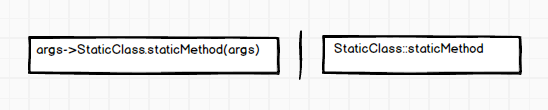Consumer<String> c1 = r -> Integer.parseInt(r);
c1.accept("1");
Consumer<String> c2 =Integer::parseInt;
c1.accept("2");


2. 实例方法引用

如果函数式接口的实现恰好可以通过调用一个实例方法来实现，那么就可以使用实例方法引用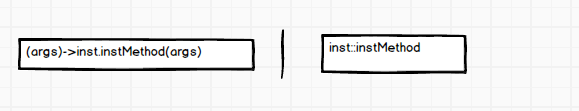Consumer<String> ins1 = r -> System.out.print(r);
c1.accept("1");
Consumer<String> ins2 =System.out::print;
c1.accept("2");


3. 对象方法引用

抽象方法的第一个参数类型刚好是实例方法的类型，抽象方法剩余的参数恰好可以当做实例方法的参数。如果函数式接口的实现能由上面说的实例方法调用来实现的话，那么就可以使用对象方法的引用。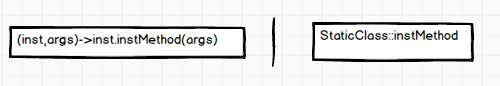Function<BigDecimal,Double> fuc1=t->t.doubleValue();
fuc1.apply(new BigDecimal("1.025"));
Function<BigDecimal,Double> fuc2=BigDecimal::doubleValue;
fuc2.apply(new BigDecimal("1.025"));

BiFunction<BigDecimal, BigDecimal, BigDecimal> func3 = (x, y) -> x.add(y);
func3.apply(new BigDecimal("1.025"), new BigDecimal("1.254"));
BiFunction<BigDecimal, BigDecimal, BigDecimal> func4 = BigDecimal::add;
func4.apply(new BigDecimal("1.025"), new BigDecimal("1.254"));


4. 构造方法引用

如果函数式接口的实现恰好可以通过调用一个类的构造方法来实现，那么就可以使用构造方法引用。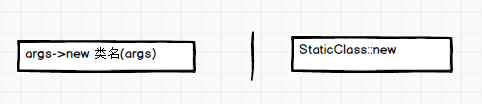Consumer<String> n1 = r ->new BigDecimal(r);
c1.accept("1");
Consumer<String> n2 =BigDecimal::new;
c1.accept("2");


stream API的引用

Stream是处理数组和集合的API,Stream具有以下特点：

• 不是数据结构，没有内部存储
• 不支持索引访问
• 延迟计算
• 支持过滤，查找，转换，汇总等操作

   Stream st=Arrays.asList(1,2,3,4,5).stream().filter(x->{
System.out.print(x);
return  x>3;
});


   Stream st=Arrays.asList(1,2,3,4,5).stream().filter(x->{
System.out.print(x);
return  x>3;
});

st.forEach(t-> System.out.print(t));



1234455



中间操作：

• 过滤 filter
• 去重 distinct
• 排序 sorted
• 截取 limit、skip
• 转换 map/flatMap
• 其他 peek

终止操作

• 循环 forEach
• 计算 min、max、count、 average
• 匹配 anyMatch、 allMatch、 noneMatch、 findFirst、 findAny
• 汇聚 reduce
• 收集器 toArray collect

常用的几个lambda

  public class Student {

public Student(String stuName, int age, BigDecimal score, int clazz) {
this.stuName = stuName;
this.age = age;
this.score = score;
this.clazz = clazz;
}

private String stuName;
private int age;
private BigDecimal score;
private int clazz;

public String getStuName() {
return stuName;
}
public void setStuName(String stuName) {
this.stuName = stuName;
}
public int getAge() {
return age;
}
public void setAge(int age) {
this.age = age;
}
public BigDecimal getScore() {
return score;
}
public void setScore(BigDecimal score) {
this.score = score;
}
public int getClazz() {
return clazz;
}
public void setClazz(int clazz) {
this.clazz = clazz;
}
}

List<Student> studentList = new ArrayList<>();
studentList.add(new Student("黎  明", 20, new BigDecimal(80), 1));
studentList.add(new Student("郭敬明", 22, new BigDecimal(90), 2));
studentList.add(new Student("明  道", 21, new BigDecimal(65.5), 3));
studentList.add(new Student("郭富城", 30, new BigDecimal(90.5), 4));
studentList.add(new Student("刘诗诗", 20, new BigDecimal(75), 1));
studentList.add(new Student("成  龙", 60, new BigDecimal(88), 5));
studentList.add(new Student("郑伊健", 60, new BigDecimal(86), 1));
studentList.add(new Student("刘德华", 40, new BigDecimal(81), 1));
studentList.add(new Student("古天乐", 50, new BigDecimal(83), 2));
studentList.add(new Student("赵文卓", 40, new BigDecimal(84), 2));
studentList.add(new Student("吴奇隆", 30, new BigDecimal(86), 4));
studentList.add(new Student("言承旭", 50, new BigDecimal(68), 1));
studentList.add(new Student("郑伊健", 60, new BigDecimal(86), 1));
studentList.add(new Student("黎  明", 20, new BigDecimal(80), 1));
studentList.add(new Student("李连杰", 65, new BigDecimal(86), 4));
studentList.add(new Student("周润发", 69, new BigDecimal(58), 1));
studentList.add(new Student("徐若萱", 28, new BigDecimal(88), 6));
studentList.add(new Student("许慧欣", 26, new BigDecimal(86), 8));
studentList.add(new Student("陈慧琳", 35, new BigDecimal(64), 1));
studentList.add(new Student("关之琳", 45, new BigDecimal(50), 9));
studentList.add(new Student("温碧霞", 67, new BigDecimal(53), 2));
studentList.add(new Student("林青霞", 22, new BigDecimal(56), 3));
studentList.add(new Student("李嘉欣", 25, new BigDecimal(84), 1));
studentList.add(new Student("彭佳慧", 26, new BigDecimal(82), 5));
studentList.add(new Student("陈紫涵", 39, new BigDecimal(88), 1));
studentList.add(new Student("张韶涵", 41, new BigDecimal(90), 6));
studentList.add(new Student("梁朝伟", 58, new BigDecimal(74), 1));
studentList.add(new Student("梁咏琪", 65, new BigDecimal(82), 7));
studentList.add(new Student("范玮琪", 22, new BigDecimal(83), 1));



forEach

forEach:代表循环当前的list ,下面的例子是循环打印出student的名字


studentList.stream().forEach(x -> System.out.println(x.getStuName()));



filter


studentList.stream().filter(t -> t.getScore().compareTo(new BigDecimal(80)) > 0).forEach(x -> System.out.println(x.getStuName()));



distinct、sorted 、group

1. 去除重复数据


studentList.stream().distinct().forEach(x -> System.out.println(x.getStuName()));


2. 单条件排序和多条件排序


studentList.stream().sorted(Comparator.comparing(Student::getScore)).forEach(x -> System.out.println(x.getStuName()));

//多条件排序
studentList.stream().sorted(Comparator.comparing(Student::getScore).thenComparing(Student::getStuName)).forEach(x -> System.out.println(x.getStuName()));


3. group 的使用


System.out.println(studentList.stream().collect(Collectors.groupingBy(x->x.getAge(),Collectors.counting())));



limit、skip


studentList.stream().skip(10).limit(5).forEach(x -> System.out.println(x.getStuName()));

//具体的分页
int pageIndex=1;
int pageSize=5;
studentList.stream().skip((pageIndex-1)*pageSize).limit(pageSize).forEach(x -> System.out.println(x.getStuName()));



map/flatMap

map是一个转换的工具，提供很多转换的方法，mapToInt,mapToDouble


studentList.stream().map(Student::getScore).forEach(x -> System.out.println(x));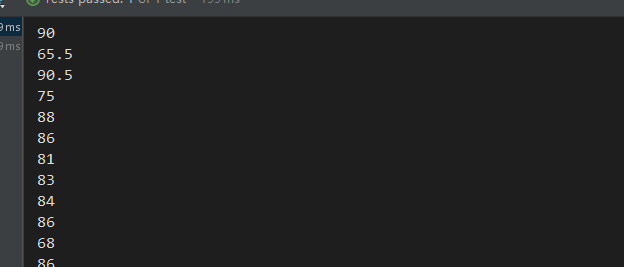flatMap是一个可以把子数组的值放到数组里面, 下面的实例是把所有的名字都拆开成一个新的数组


studentList.stream().flatMap(x-> Arrays.stream(x.getStuName().split(""))).forEach(x -> System.out.println(x));min、max、count、 average


studentList.stream().max(Comparator.comparing(x -> x.getAge())).ifPresent(x-> System.out.println(x.getAge()));
studentList.stream().min(Comparator.comparing(x -> x.getAge())).ifPresent(x-> System.out.println(x.getAge()));
System.out.println(studentList.stream().count());
studentList.stream().mapToDouble(x -> x.getScore().doubleValue()).average().ifPresent(x-> System.out.println(x));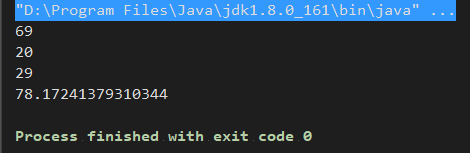anyMatch、noneMatch、 allMatch、 findFirst、 findAny

• anyMatch： 操作用于判断Stream中的是否有满足指定条件的元素。如果最少有一个满足条件返回true，否则返回false。

• noneMatch： 与anyMatch相反。allMatch是判断所有元素是不是都满足表达式。

• findFirst： 操作用于获取含有Stream中的第一个元素的Optional，如果Stream为空，则返回一个空的Optional。若Stream并未排序，可能返回含有Stream中任意元素的Optional。

• findAny： 操作用于获取含有Stream中的某个元素的Optional，如果Stream为空，则返回一个空的Optional。由于此操作的行动是不确定的，其会自由的选择Stream中的任何元素。在并行操作中，在同一个Stram中多次调用，可能会不同的结果。在串行调用时，都是获取的第一个元素， 默认的是获取第一个元素,并行是随机的返回。


System.out.println(studentList.stream().anyMatch(r -> r.getStuName().contains("伟")));
System.out.println(studentList.stream().allMatch(r -> r.getStuName().contains("伟")));
System.out.println(studentList.stream().noneMatch(r -> r.getStuName().contains("伟")));
System.out.println(studentList.stream().findFirst());
System.out.println(studentList.stream().findAny());

for (int i=0;i<10;i++)
{
System.out.println(studentList.stream().parallel().findAny().get().getStuName());
}



reduce


Stream.of(1, 5, 10, 8).reduce((x, y) -> {
System.out.println("x : " + x);
System.out.println("y : " + y);
System.out.println("x+y : " +x);

System.out.println("--------");
return x + y;
});



x : 1
y : 5
x+y : 1
--------
x : 6
y : 10
x+y : 6
--------
x : 16
y : 8
x+y : 16
--------



1. reduce是一个循环，有两个参数
2. 第一次执行的时候x是第一个值，y是第二个值。
3. 在第二次执行的时候，x是上次返回的值，y是第三个值
…. 直到循环结束为止。


//指定了初始值

Stream.of(1, 5, 10, 8).reduce(100,(x, y) -> {
System.out.println("x : " + x);
System.out.println("y : " + y);
System.out.println("x+y : " +x);
System.out.println("--------");
return x + y;
});


x : 100
y : 1
x+y : 100
--------
x : 101
y : 5
x+y : 101
--------
x : 106
y : 10
x+y : 106
--------
x : 116
y : 8
x+y : 116
--------



toArray、collect

toArray和collect是两个收集器，toArray是把数据转换成数组，collect是转成其他的类型。这里就不在讨论了。


System.out.println(studentList.stream().collect(Collectors.groupingBy(x->x.getAge(),Collectors.counting())));



(本文完)